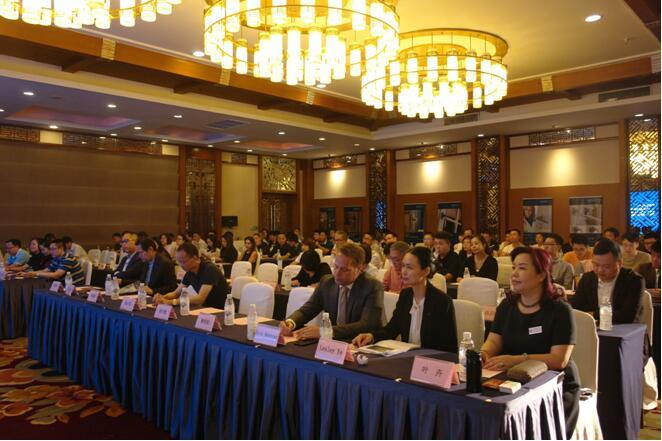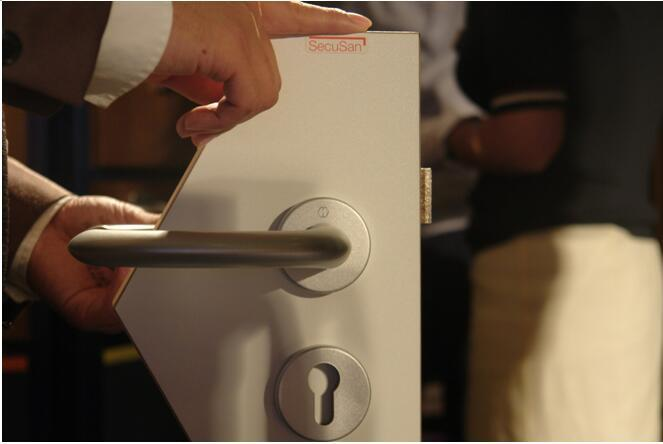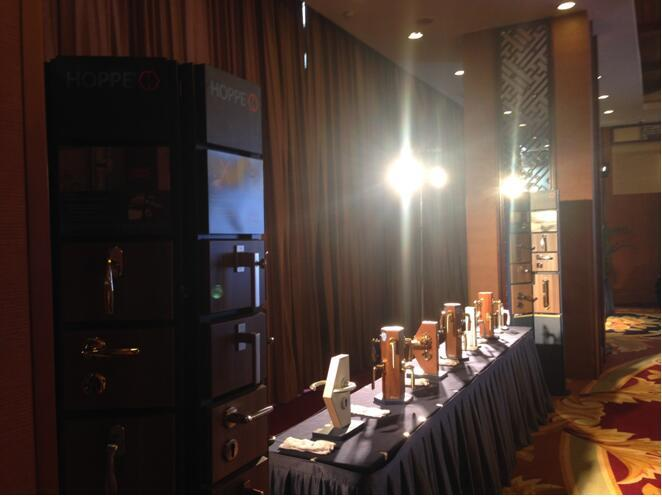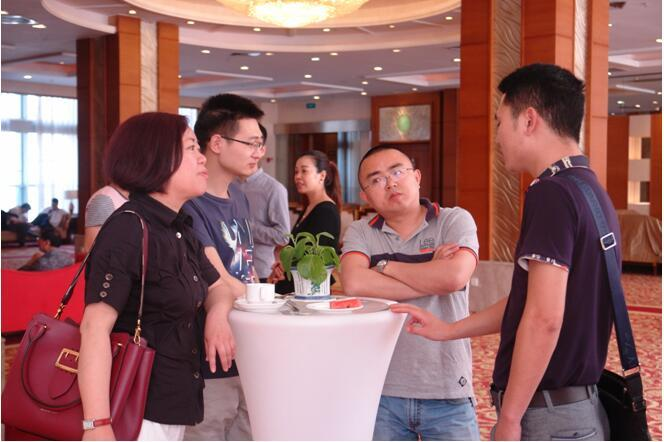|

# 好把手 博天下— 德国好博品牌推广会顺利举行

2017年5月18日，由HOPPE德国好博集团在中国的全资子公司，博恬(上海)五金有限公司主办，成都恒圆建材有限公司承办的“好把手，博天下”HOPPE德国好博品牌推广会在成都洲际大酒店国际会议中心隆重举行。推广会现场

出席会议的领导嘉宾有HOPPE德国好博亚太地区销售与市场主管Eric Kersten先生，成都恒圆建材有限公司总经理叶卉女士，行业协会领导、专家，华润置地、万华地产、和记黄埔地产、成都阳明房地产、晟丰地产、朗基地产、龙湖地产、蓝光、成都长虹置业、鼎康置业等地产公司老总，中建西南院、四川世纪创美设计、山鼎设计等设计院领导以及HOPPE德国好博的合作客户，近120人。会议全程由成都恒圆建材有限公司行政总监刘治愈女士主持。成都恒圆建材有限公司叶卉总经理致欢迎词

大会上，叶卉总经理代表“德国好博”在四川及重庆地区的指定经销商，也是本次活动的承办单位成都恒圆建材有限公司致欢迎辞，叶总首先感谢了莅临现场的行业领导、专家、房地产公司、设计院的的领导及国内外商家代表，介绍了HOPPE好博集团“意大利设计、德国工艺、瑞士管理”的先进企业管理方式和专注产品，以客户满意为导向的运营理念。同时在致辞中，叶总为来宾们阐述了恒圆公司的不断进取和以客户为本，提供专业和完善的服务的发展理念和精神，正是因为这样的契合让HOPPE好博和恒圆携手合作。HOPPE德国好博亚太地区销售与市场主管Eric Kersten先生致辞

Eric Kersten先生发言中分享了自己对HOPPE 德国好博公司的深度了解和任亚太地区销售与市场主管期间对市场的感受和经验，用简短朴实的语言介绍了传承三代“所有者管理的家族企业”如何在六十多年中执着于门窗把手研发、生产和销售。他的言语中传递出一家以“创造满意的客户”为总任务，以“增强企业生存能力”为总目标的企业的坚定，踏实的企业文化和精神。作为一名外国友人，Eric Kersten先生熟悉中国的“十三五”计划，用一张简单的市场增长数据图片震撼了全场，处处彰显出德国优秀企业管理者的风范。

伴随着Eric Kersten先生精彩的演讲推广会告一段落，会议安排了在场嘉宾茶歇和产品观摩时间，大家纷纷驻足到了HOPPE德国好博产品体验区展示台前。到场嘉宾饶有兴趣的上手触摸，感受被喻为把手中“BBA”产品的魅力，感受到德系制造严谨、专业的态度和最终呈现在产品每一个细节上的精致稳定。HOPPE德国好博工作人员现场讲解产品HOPPE德国好博产品经理洪元超做产品介绍

洪经理感受了川味的“变脸”，临时起意用“海派清口”为来宾们讲解了HOPPE德国好博产品的技术和设计特点。什么是快装方轴？什么是安全防盗技术？什么是整体系统锁– HCS®？什么是杀菌、抗菌SecuSan®技术？这些客户关心的问题在洪经理的发言中一一得到了解答。现场展示的HOPPE德国好博杀菌、抗菌SecuSan®

HOPPE德国好博SecuSan®——是一种特殊表面处理工艺，可以迅速、持久地抑制微生物生长。完全免维护，并可供长期使用。

HOPPE德国好博SecuStik®——防盗联动窗把手，使得那些“不速之客”们从外面撬开窗系统入室作案变得更加困难，从真正意义上提高了居家的安全性。山鼎设计院刘骏翔总经理做分享

刘总从设计与美学艺术的层面诠释了好的企业、好的产品为项目的实施和规划提供的优质便利，总结出“好把手博天下”“好品质赢天下”。四川良木道型材有限公司繆培友总经理做分享

繆总上台首先感谢了HOPPE德国好博及叶总。良木道一直以来都坚持使用HOPPE 好博把手，在市场口碑上和商业利润上都取得了不小的成就，是HOPPE德国好博这样的合作伙伴，叶总这样的朋友支持他、支持良木道在门窗行业内不断转型发展、勇往直前。

伴随着繆总的感恩发言，本次HOPPE德国好博品牌推广会圆满落幕，会后主办方为广大的领导、来宾准备了晚宴和详细交流的环节。

“好把手博天下”HOPPE德国好博品牌推广会掠影：叶卉女士与Eric Kersten先生合影HOPPE德国好博产品现场展示与会嘉宾茶歇交流

转自中国幕墙网

`声明：本文由入驻焦点开放平台的作者撰写，除焦点官方账号外，观点仅代表作者本人，不代表焦点立场错误信息举报电话： 400-099-0099，邮箱：jubao@vip.sohu.com，或点此进行意见反馈，或点此进行举报投诉。`A B C D E F G H J K L M N P Q R S T W X Y Z
A - B - C - D - E
• A
• 鞍山
• 安庆
• 安阳
• 安顺
• 安康
• 澳门
• B
• 北京
• 保定
• 包头
• 巴彦淖尔
• 本溪
• 蚌埠
• 亳州
• 滨州
• 北海
• 百色
• 巴中
• 毕节
• 保山
• 宝鸡
• 白银
• 巴州
• C
• 承德
• 沧州
• 长治
• 赤峰
• 朝阳
• 长春
• 常州
• 滁州
• 池州
• 长沙
• 常德
• 郴州
• 潮州
• 崇左
• 重庆
• 成都
• 楚雄
• 昌都
• 慈溪
• 常熟
• D
• 大同
• 大连
• 丹东
• 大庆
• 东营
• 德州
• 东莞
• 德阳
• 达州
• 大理
• 德宏
• 定西
• 儋州
• 东平
• E
• 鄂尔多斯
• 鄂州
• 恩施
F - G - H - I - J
• F
• 抚顺
• 阜新
• 阜阳
• 福州
• 抚州
• 佛山
• 防城港
• G
• 赣州
• 广州
• 桂林
• 贵港
• 广元
• 广安
• 贵阳
• 固原
• H
• 邯郸
• 衡水
• 呼和浩特
• 呼伦贝尔
• 葫芦岛
• 哈尔滨
• 黑河
• 淮安
• 杭州
• 湖州
• 合肥
• 淮南
• 淮北
• 黄山
• 菏泽
• 鹤壁
• 黄石
• 黄冈
• 衡阳
• 怀化
• 惠州
• 河源
• 贺州
• 河池
• 海口
• 红河
• 汉中
• 海东
• I
• J
• 晋中
• 锦州
• 吉林
• 鸡西
• 佳木斯
• 嘉兴
• 金华
• 景德镇
• 九江
• 吉安
• 济南
• 济宁
• 焦作
• 荆门
• 荆州
• 江门
• 揭阳
• 金昌
• 酒泉
• 嘉峪关
K - L - M - N - P
• K
• 开封
• 昆明
• 昆山
• L
• 廊坊
• 临汾
• 辽阳
• 连云港
• 丽水
• 六安
• 龙岩
• 莱芜
• 临沂
• 聊城
• 洛阳
• 漯河
• 娄底
• 柳州
• 来宾
• 泸州
• 乐山
• 六盘水
• 丽江
• 临沧
• 拉萨
• 林芝
• 兰州
• 陇南
• M
• 牡丹江
• 马鞍山
• 茂名
• 梅州
• 绵阳
• 眉山
• N
• 南京
• 南通
• 宁波
• 南平
• 宁德
• 南昌
• 南阳
• 南宁
• 内江
• 南充
• P
• 盘锦
• 莆田
• 平顶山
• 濮阳
• 攀枝花
• 普洱
• 平凉
Q - R - S - T - W
• Q
• 秦皇岛
• 齐齐哈尔
• 衢州
• 泉州
• 青岛
• 清远
• 钦州
• 黔南
• 曲靖
• 庆阳
• R
• 日照
• 日喀则
• S
• 石家庄
• 沈阳
• 双鸭山
• 绥化
• 上海
• 苏州
• 宿迁
• 绍兴
• 宿州
• 三明
• 上饶
• 三门峡
• 商丘
• 十堰
• 随州
• 邵阳
• 韶关
• 深圳
• 汕头
• 汕尾
• 三亚
• 三沙
• 遂宁
• 山南
• 商洛
• 石嘴山
• T
• 天津
• 唐山
• 太原
• 通辽
• 铁岭
• 泰州
• 台州
• 铜陵
• 泰安
• 铜仁
• 铜川
• 天水
• 天门
• W
• 乌海
• 乌兰察布
• 无锡
• 温州
• 芜湖
• 潍坊
• 威海
• 武汉
• 梧州
• 渭南
• 武威
• 吴忠
• 乌鲁木齐
X - Y - Z
• X
• 邢台
• 徐州
• 宣城
• 厦门
• 新乡
• 许昌
• 信阳
• 襄阳
• 孝感
• 咸宁
• 湘潭
• 湘西
• 西双版纳
• 西安
• 咸阳
• 西宁
• 仙桃
• 西昌
• Y
• 运城
• 营口
• 盐城
• 扬州
• 鹰潭
• 宜春
• 烟台
• 宜昌
• 岳阳
• 益阳
• 永州
• 阳江
• 云浮
• 玉林
• 宜宾
• 雅安
• 玉溪
• 延安
• 榆林
• 银川
• Z
• 张家口
• 镇江
• 舟山
• 漳州
• 淄博
• 枣庄
• 郑州
• 周口
• 驻马店
• 株洲
• 张家界
• 珠海
• 湛江
• 肇庆
• 中山
• 自贡
• 资阳
• 遵义
• 昭通
• 张掖
• 中卫

1室1厅1厨1卫1阳台

1
2
3
4
5

0
1
2

1

1

0
1
2
3报名成功，资料已提交审核A B C D E F G H J K L M N P Q R S T W X Y Z
A - B - C - D - E
• A
• 鞍山
• 安庆
• 安阳
• 安顺
• 安康
• 澳门
• B
• 北京
• 保定
• 包头
• 巴彦淖尔
• 本溪
• 蚌埠
• 亳州
• 滨州
• 北海
• 百色
• 巴中
• 毕节
• 保山
• 宝鸡
• 白银
• 巴州
• C
• 承德
• 沧州
• 长治
• 赤峰
• 朝阳
• 长春
• 常州
• 滁州
• 池州
• 长沙
• 常德
• 郴州
• 潮州
• 崇左
• 重庆
• 成都
• 楚雄
• 昌都
• 慈溪
• 常熟
• D
• 大同
• 大连
• 丹东
• 大庆
• 东营
• 德州
• 东莞
• 德阳
• 达州
• 大理
• 德宏
• 定西
• 儋州
• 东平
• E
• 鄂尔多斯
• 鄂州
• 恩施
F - G - H - I - J
• F
• 抚顺
• 阜新
• 阜阳
• 福州
• 抚州
• 佛山
• 防城港
• G
• 赣州
• 广州
• 桂林
• 贵港
• 广元
• 广安
• 贵阳
• 固原
• H
• 邯郸
• 衡水
• 呼和浩特
• 呼伦贝尔
• 葫芦岛
• 哈尔滨
• 黑河
• 淮安
• 杭州
• 湖州
• 合肥
• 淮南
• 淮北
• 黄山
• 菏泽
• 鹤壁
• 黄石
• 黄冈
• 衡阳
• 怀化
• 惠州
• 河源
• 贺州
• 河池
• 海口
• 红河
• 汉中
• 海东
• I
• J
• 晋中
• 锦州
• 吉林
• 鸡西
• 佳木斯
• 嘉兴
• 金华
• 景德镇
• 九江
• 吉安
• 济南
• 济宁
• 焦作
• 荆门
• 荆州
• 江门
• 揭阳
• 金昌
• 酒泉
• 嘉峪关
K - L - M - N - P
• K
• 开封
• 昆明
• 昆山
• L
• 廊坊
• 临汾
• 辽阳
• 连云港
• 丽水
• 六安
• 龙岩
• 莱芜
• 临沂
• 聊城
• 洛阳
• 漯河
• 娄底
• 柳州
• 来宾
• 泸州
• 乐山
• 六盘水
• 丽江
• 临沧
• 拉萨
• 林芝
• 兰州
• 陇南
• M
• 牡丹江
• 马鞍山
• 茂名
• 梅州
• 绵阳
• 眉山
• N
• 南京
• 南通
• 宁波
• 南平
• 宁德
• 南昌
• 南阳
• 南宁
• 内江
• 南充
• P
• 盘锦
• 莆田
• 平顶山
• 濮阳
• 攀枝花
• 普洱
• 平凉
Q - R - S - T - W
• Q
• 秦皇岛
• 齐齐哈尔
• 衢州
• 泉州
• 青岛
• 清远
• 钦州
• 黔南
• 曲靖
• 庆阳
• R
• 日照
• 日喀则
• S
• 石家庄
• 沈阳
• 双鸭山
• 绥化
• 上海
• 苏州
• 宿迁
• 绍兴
• 宿州
• 三明
• 上饶
• 三门峡
• 商丘
• 十堰
• 随州
• 邵阳
• 韶关
• 深圳
• 汕头
• 汕尾
• 三亚
• 三沙
• 遂宁
• 山南
• 商洛
• 石嘴山
• T
• 天津
• 唐山
• 太原
• 通辽
• 铁岭
• 泰州
• 台州
• 铜陵
• 泰安
• 铜仁
• 铜川
• 天水
• 天门
• W
• 乌海
• 乌兰察布
• 无锡
• 温州
• 芜湖
• 潍坊
• 威海
• 武汉
• 梧州
• 渭南
• 武威
• 吴忠
• 乌鲁木齐
X - Y - Z
• X
• 邢台
• 徐州
• 宣城
• 厦门
• 新乡
• 许昌
• 信阳
• 襄阳
• 孝感
• 咸宁
• 湘潭
• 湘西
• 西双版纳
• 西安
• 咸阳
• 西宁
• 仙桃
• 西昌
• Y
• 运城
• 营口
• 盐城
• 扬州
• 鹰潭
• 宜春
• 烟台
• 宜昌
• 岳阳
• 益阳
• 永州
• 阳江
• 云浮
• 玉林
• 宜宾
• 雅安
• 玉溪
• 延安
• 榆林
• 银川
• Z
• 张家口
• 镇江
• 舟山
• 漳州
• 淄博
• 枣庄
• 郑州
• 周口
• 驻马店
• 株洲
• 张家界
• 珠海
• 湛江
• 肇庆
• 中山
• 自贡
• 资阳
• 遵义
• 昭通
• 张掖
• 中卫• 手机• 分享
• 设计
免费设计
• 计算器
装修计算器
• 入驻
合作入驻
• 联系
联系我们
• 置顶
返回顶部